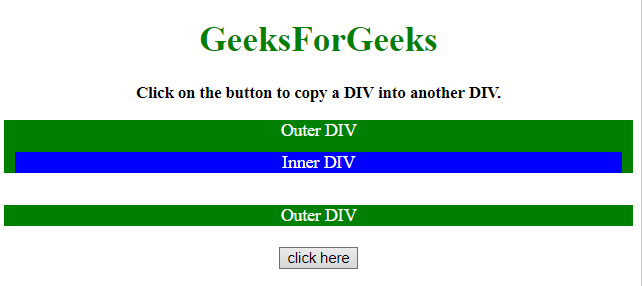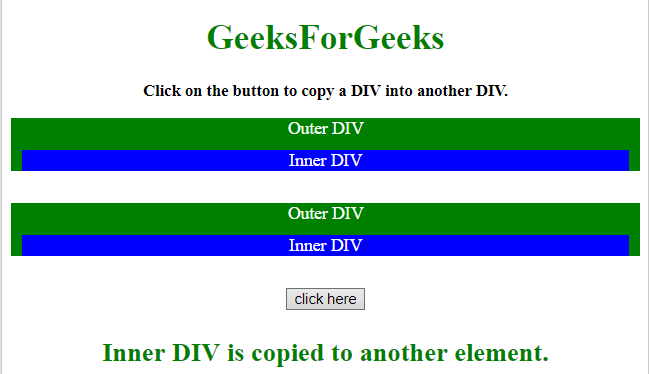# How to copy the content of a div into another div using jQuery ?

Given an HTML document containing some div element and the task is to copy a div content into another div as its child using jQuery. There are two approaches to solve this problem which are discussed below:

Approach 1:

• First, select the div element which need to be copy into another div element.
• Select the target element where div eleemtn is copied.
• Use the append() method to copy the element as its child.

Example: This example uses append() method to copy the div element into another div.

 `   ` `<``html``>   ` ` `  `<``head``>   ` `    ``<``title``>   ` `        ``How to copy the content of a div  ` `        ``into another div using jQuery ? ` `    `` ` `     `  `    ``<``script` `src``= ` `"https://ajax.googleapis.com/ajax/libs/jquery/3.4.1/jquery.min.js"``> ` `    `` ` `     `  `    ``<``style``> ` `        ``.parent { ` `            ``background: green; ` `            ``color: white; ` `        ``} ` `        ``.child { ` `            ``background: blue; ` `            ``color: white; ` `            ``margin: 10px; ` `        ``}   ` `        ``#GFG_UP { ` `            ``font-size: 15px; ` `            ``font-weight: bold; ` `        ``} ` `        ``#GFG_DOWN { ` `            ``font-size: 24px; ` `            ``font-weight: bold; ` `            ``color: green; ` `        ``} ` `    `` ` `   ` ` `  `<``body` `id` `= ``"body"` `style` `= ``"text-align:center;"``>   ` `     `  `    ``<``h1` `style` `= ``"color:green;"` `>   ` `        ``GeeksForGeeks   ` `    ``  ` `     `  `    ``<``p` `id` `= ``"GFG_UP"``> ` `     `  `    ``<``div` `class``=``"parent"``> ` `        ``Outer DIV ` `        ``<``div` `class``=``"child"``> ` `            ``Inner DIV ` `        `` ` `    `` ` `     `  `    ``<``br``> ` `     `  `    ``<``div` `class``=``"parent"` `id` `= ``"parent2"``> ` `        ``Outer DIV ` `    `` ` `    ``<``br``> ` `     `  `    ``<``button` `onclick` `= ``"GFG_Fun()"``> ` `        ``click here ` `    `` ` `     `  `    ``<``p` `id` `= ``"GFG_DOWN"``> ` `     `  `    ``<``script``>   ` `        ``var up = document.getElementById('GFG_UP'); ` `        ``var down = document.getElementById('GFG_DOWN'); ` `        ``up.innerHTML = "Click on the button to " ` `                ``+ "copy a DIV into another DIV.";  ` `         `  `        ``function GFG_Fun() { ` `            ``var \$el = \$('.child').clone(); ` `            ``\$('#parent2').append(\$el); ` `            ``down.innerHTML = "Inner DIV is copied " ` `                    ``+ "to another element."; ` `        ``}  ` `    ``   ` `   ` ` `  ` `

Output:

• Before clicking on the button:• After clicking on the button:Approach 2:

• First, select the div element which need to be copy into another div element.
• Select the target element where div eleemtn is copied.
• Use the appendTo() method to copy the element as its child.

Example: This example uses appendTo() method to copy the div element into another div.

 `   ` `<``html``>   ` ` `  `<``head``>   ` `    ``<``title``>   ` `        ``How to copy the content of a div  ` `        ``into another div using jQuery ? ` `    `` ` `     `  `    ``<``script` `src``= ` `"https://ajax.googleapis.com/ajax/libs/jquery/3.4.1/jquery.min.js"``> ` `    `` ` `     `  `    ``<``style``> ` `        ``.parent { ` `            ``background: green; ` `            ``color: white; ` `        ``} ` `        ``.child { ` `            ``background: blue; ` `            ``color: white; ` `            ``margin: 10px; ` `        ``}   ` `        ``#GFG_UP { ` `            ``font-size: 15px; ` `            ``font-weight: bold; ` `        ``} ` `        ``#GFG_DOWN { ` `            ``font-size: 24px; ` `            ``font-weight: bold; ` `            ``color: green; ` `        ``} ` `    `` ` `   ` ` `  `<``body` `id` `= ``"body"` `style` `= ``"text-align:center;"``>   ` `     `  `    ``<``h1` `style` `= ``"color:green;"` `>   ` `        ``GeeksForGeeks   ` `    ``  ` `     `  `    ``<``p` `id` `= ``"GFG_UP"``> ` `     `  `    ``<``div` `class``=``"parent"``> ` `        ``Outer DIV ` `        ``<``div` `class``=``"child"``> ` `            ``Inner DIV ` `        `` ` `    `` ` `     `  `    ``<``br``> ` `     `  `    ``<``div` `class``=``"parent"` `id` `= ``"parent2"``> ` `        ``Outer DIV ` `    `` ` `    ``<``br``> ` `     `  `    ``<``button` `onclick` `= ``"GFG_Fun()"``> ` `        ``click here ` `    `` ` `     `  `    ``<``p` `id` `= ``"GFG_DOWN"``> ` `     `  `    ``<``script``>   ` `        ``var up = document.getElementById('GFG_UP'); ` `        ``var down = document.getElementById('GFG_DOWN'); ` `        ``up.innerHTML = "Click on the button to " ` `                ``+ "copy a DIV into another DIV.";  ` `         `  `        ``function GFG_Fun() { ` `            ``\$('.child').clone().appendTo('#parent2'); ` `            ``down.innerHTML = "Inner DIV is copied" ` `                        ``+ " to another element."; ` `        ``}  ` `    ``   ` `   ` ` `  ` `

Output:

• Before clicking on the button:• After clicking on the button:My Personal Notes arrow_drop_upCheck out this Author's contributed articles.

If you like GeeksforGeeks and would like to contribute, you can also write an article using contribute.geeksforgeeks.org or mail your article to contribute@geeksforgeeks.org. See your article appearing on the GeeksforGeeks main page and help other Geeks.

Please Improve this article if you find anything incorrect by clicking on the "Improve Article" button below.